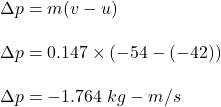## A baseball has mass 0.147 kg. If the velocity of a pitched ball has a magnitude of 42.0 m/s and the batted ball’s velocity is 54.0 m/s in th

Question

A baseball has mass 0.147 kg. If the velocity of a pitched ball has a magnitude of 42.0 m/s and the batted ball’s velocity is 54.0 m/s in the opposite direction, find the magnitude of the change in momentum of the ball and of the impulse applied to it by the bat.

in progress 0
2 weeks 2021-08-29T18:55:29+00:00 1 Answers 0 views 0

1. Explanation:

Given that,

Mass of the baseball, m = 0.147 kg

The initial speed of the baseball, u = 42 m/s

The final speed of the baseball, v = -54 m/s (in opposite direction)

We need to find the magnitude of the change in momentum of the ball and of the impulse applied to it by the bat. The change in momentum is given by :We know that the impulse applied to it by the bat is equal to the change in momentum. So, the impulse is 1.764 kg-m/s.